# Python:感知器 (五十七)

## 感知器技巧 - 计算机如何“学习”分类？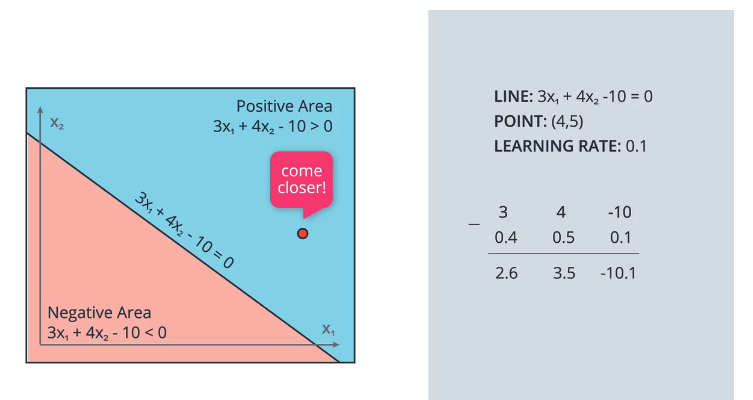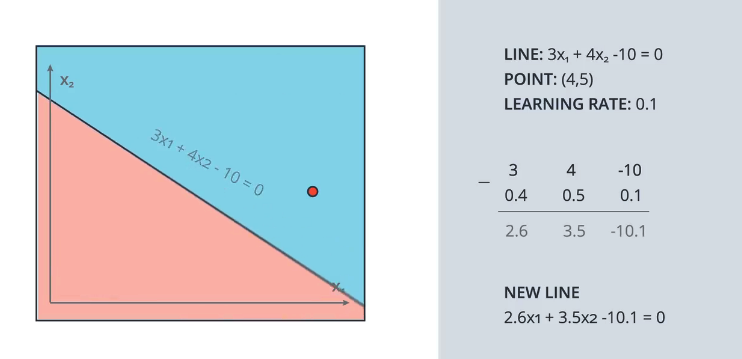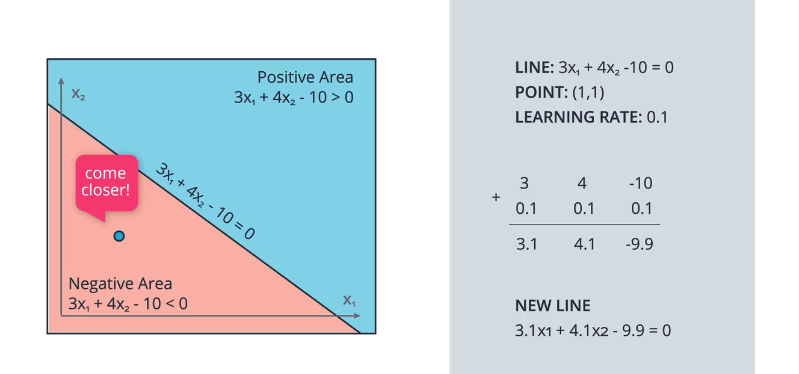## 感知器算法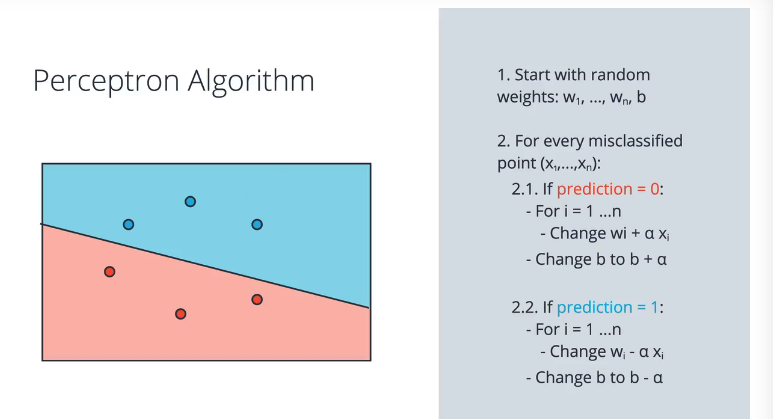### 编写感知器算法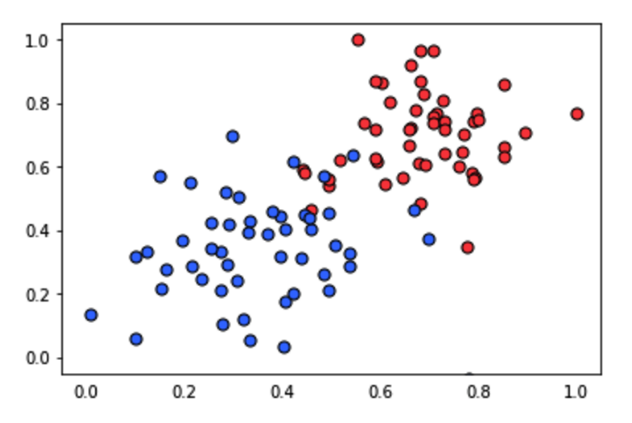• 如果点分类正确，则什么也不做。
• 如果点分类为正，但是标签为负，则分别减去 αp,αq, 和 α 至 $w_1, w_2$ , 和 b
• 如果点分类为负，但是标签为正，则分别将 αp,αq, 和 α 加到 w_1, w_2, w , 和 b 上。

import numpy as np
# Setting the random seed, feel free to change it and see different solutions.
np.random.seed(42)

def stepFunction(t):
if t >= 0:
return 1
return 0

def prediction(X, W, b):
return stepFunction((np.matmul(X,W)+b))

# TODO: Fill in the code below to implement the perceptron trick.
# The function should receive as inputs the data X, the labels y,
# the weights W (as an array), and the bias b,
# update the weights and bias W, b, according to the perceptron algorithm,
# and return W and b.
def perceptronStep(X, y, W, b, learn_rate = 0.01):
for i in range(len(X)):
y_hat = prediction(X[i],W,b)
if y[i]-y_hat == 1:
W += X[i]*learn_rate
W += X[i]*learn_rate
b += learn_rate
elif y[i]-y_hat == -1:
W -= X[i]*learn_rate
W -= X[i]*learn_rate
b -= learn_rate
return W, b

# This function runs the perceptron algorithm repeatedly on the dataset,
# and returns a few of the boundary lines obtained in the iterations,
# for plotting purposes.
# Feel free to play with the learning rate and the num_epochs,
# and see your results plotted below.
def trainPerceptronAlgorithm(X, y, learn_rate = 0.01, num_epochs = 25):
x_min, x_max = min(X.T), max(X.T)
y_min, y_max = min(X.T), max(X.T)
W = np.array(np.random.rand(2,1))
b = np.random.rand(1) + x_max
# These are the solution lines that get plotted below.
boundary_lines = []
for i in range(num_epochs):
# In each epoch, we apply the perceptron step.
W, b = perceptronStep(X, y, W, b, learn_rate)
boundary_lines.append((-W/W, -b/W))
return boundary_lines


## 非线性界线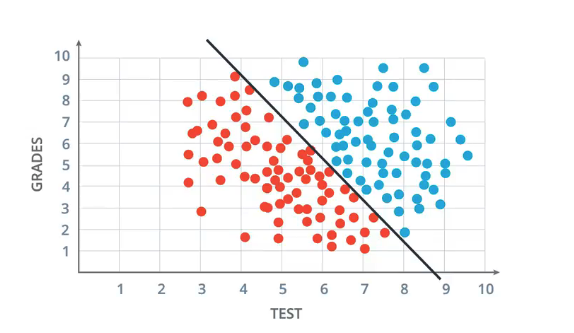## 误差函数

An error function is simply something that tells us how far we are from the solution.

## 误差函数与梯度下降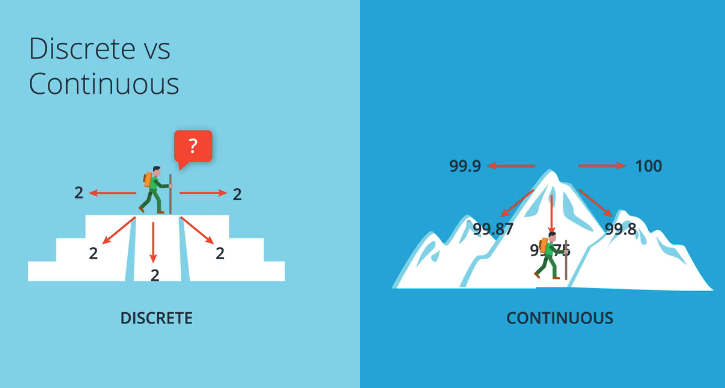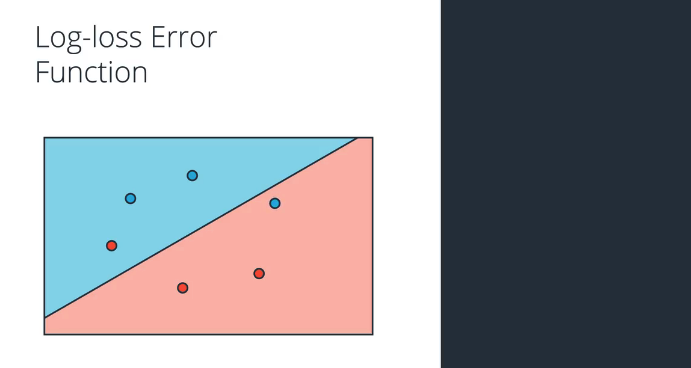### Gradient Descent(梯度下降)